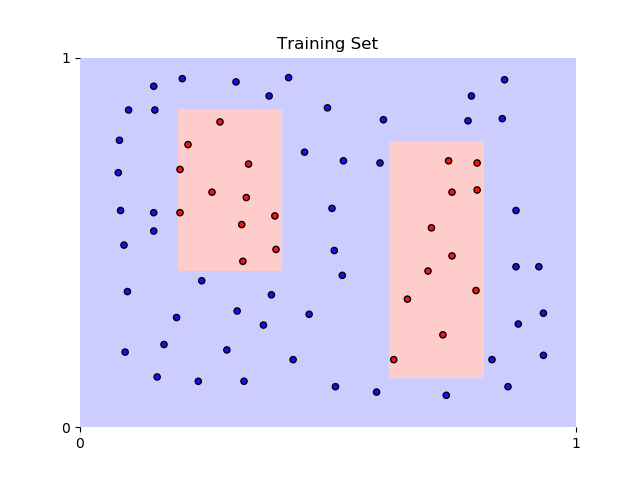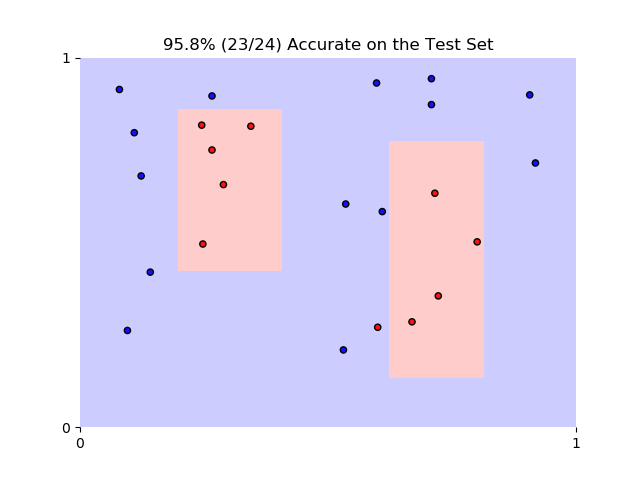# Visualizing Decision Boundaries

### Train Test Split

# Import statements
from sklearn.tree import DecisionTreeClassifier
from sklearn.metrics import accuracy_score
import pandas as pd
import numpy as np

# Import the train test split
from sklearn.model_selection import train_test_split

# Read in the data


0 1 2
0 0.24539 0.81725 0
1 0.21774 0.76462 0
2 0.20161 0.69737 0
3 0.20161 0.58041 0
4 0.24770 0.49561 0
data = np.asarray(df)

# Assign the features to the variable X, and the labels to the variable y
X = data[:,0:2]
y = data[:,2]

# Use train test split to split your data
X_train, X_test, y_train, y_test = train_test_split(X, y, random_state=42)

# Instantiate the model and fit it to the training data
model = DecisionTreeClassifier().fit(X_train, y_train)

# Use the trained model to make predictions
y_pred = model.predict(X_test)

# Calculate the accuracy and assign it to the variable acc on the test data
acc_percent = accuracy_score(y_pred, y_test)
acc_numerator = accuracy_score(y_pred, y_test, normalize=False)
accuracy_string = '{:2.1f}% ({}/{}) Accurate on the Test Set'.format(acc_percent*100.,
acc_numerator,
y_test.shape)
print(accuracy_string)

95.8% (23/24) Accurate on the Test Set


#### Visualize the Decision Boundaries

%matplotlib notebook

import matplotlib.pyplot as plt
from matplotlib.colors import ListedColormap

cmap_light = ListedColormap(['#FFCCCC', '#CCCCFF'])
cmap_bold = ListedColormap(['#FF1111', '#1111FF'])

mesh_step_size = .001
mark_symbol_size = 20

def plot_decision_boundaries(Xs, Ys, title):
plt.figure()

# Plot Decision Boundaries
xx, yy = np.meshgrid(np.arange(0, 1, mesh_step_size),
np.arange(0, 1, mesh_step_size))
Z = model.predict(np.c_[xx.ravel(),
yy.ravel()])
Z = Z.reshape(xx.shape)
plt.pcolormesh(xx, yy, Z, cmap=cmap_light)

# Plot Points
plt.scatter(Xs[:, 0],
Xs[:, 1],
s=mark_symbol_size,
c=Ys,
cmap=cmap_bold,
edgecolor='black')
plt.title(title)

for spine in plt.gca().spines.values():
spine.set_visible(False)
plt.xticks([0.0,1.0])
plt.yticks([0.0,1.0]);

plot_decision_boundaries(X_train, y_train, 'Training Set');

<IPython.core.display.Javascript object>plot_decision_boundaries(X_test, y_test, accuracy_string);

<IPython.core.display.Javascript object>